# IBPS PO Prelims Quantitative Aptitude Questions 2021 (Day-09)

Dear Aspirants, Our IBPS Guide team is providing new series of Quantitative Aptitude Questions for IBPS PO Prelims 2021 so the aspirants can practice it on a daily basis. These questions are framed by our skilled experts after understanding your needs thoroughly. Aspirants can practice these new series questions daily to familiarize with the exact exam pattern and make your preparation effective.

Start Quiz

Approximation

Directions (01-05): What approximate value should come in place of (?) in the following questions?

1) 49.97*25.03 – 28.94*10.04 = (35.99 + ?) * 10.03

A.85

B.92

C.45

D.60

E.59

2)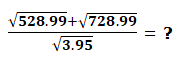A.68

B.52

C.64

D.85

E.25

3)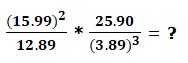A.10

B.8

C.9

D.11

E.15

4) 2499*49.85% + √1295 – 261.99 = ?²

A.35

B.48

C.62

D.32

E.58

5)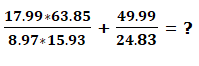A.10

B.20

C.40

D.30

E.50

Pie chart

Directions (06-10): Study the following information carefully and answer the questions given below.

The given pie chart shows the number of employees work in five different companies.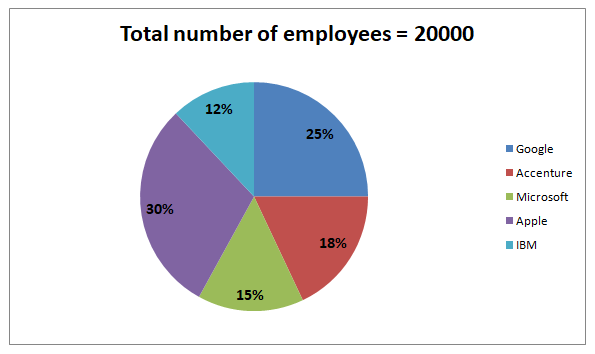6) What is the difference between the number of employees work in IBM and Microsoft?

A.400

B.500

C.600

D.700

E.900

7) What is the ratio of the average number of employees works in Google and Apple together to the average number of employees works in Microsoft and Accenture together?

A.4:3

B.5:3

C.7:3

D.3:1

E.None of these

8) What is the average number of employees work in IBM and Accenture?

A.3000

B.3200

C.3500

D.3600

E.2800

9) The number of employees in Google and Microsoft together is what percent of the number of employees in Accenture and Apple together?

A.71.42%

B.66.66%

C.83.33%

D.87.76%

E.77.77%

10) The number of employees in CTS is 20% more than the number of employees in IBM and the number of employees in TCS is 30% more than the number of employees in Microsoft. What is the difference between the number of employees in TCS and CTS?

A.1020

B.1040

C.1060

D.1080

E.1100

### Try IBPS PO Prelims Free Online Mock Test

50*25 – 29*10 = (36 + ?)*10

1250 – 290 = (36+?)*10

960 = (36+?)*10

? = 60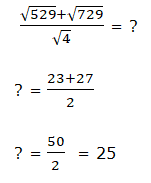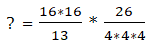? = 8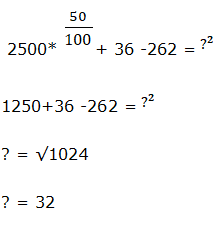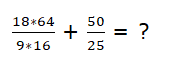? = 8 + 2 = 10

Required difference = (15 – 12)/100 * 20000

= 600

Required ratio = (25 + 30)/2:(15 + 18)/2

= 55:33

= 5:3

Required average = ((12 + 18)/2) * 20000/100 = 3000

Required percentage = (25 + 15)/(18 + 30) * 100 = 83.33%

Number of employees in CTS = 120/100 * 12/100 * 20000 = 2880

Number of employees in TCS = 130/100 * 15/100 * 20000 = 3900

Difference = 3900 – 2880 = 1020

For More Days IBPS PO Prelims Practice Set 2021

Get Quantitative Aptitude Study Materials

Get All Section Study Materials